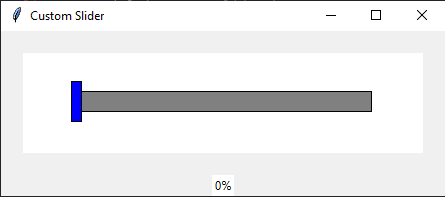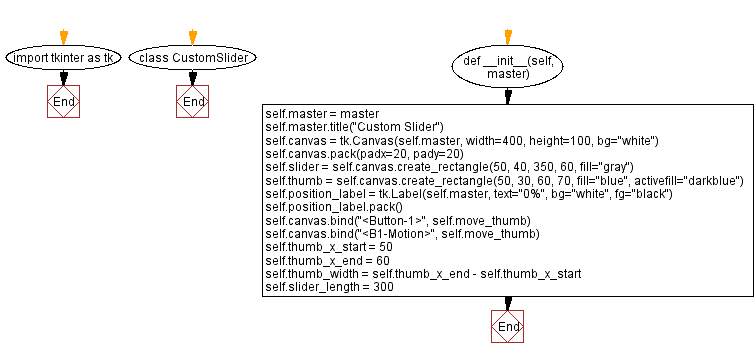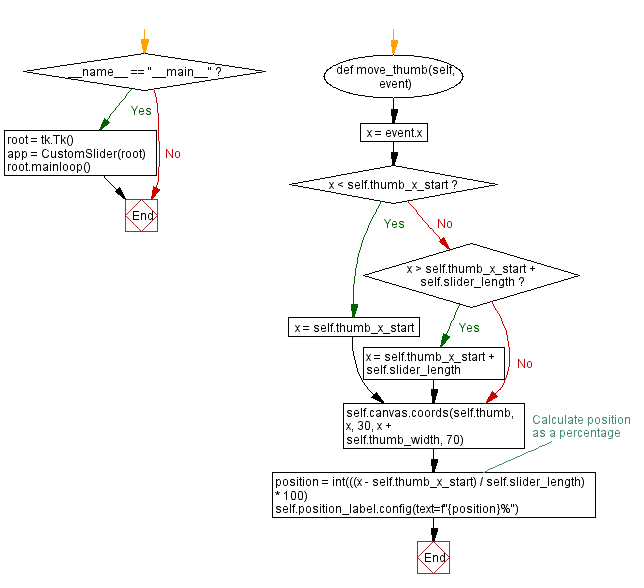﻿ Customizing Tkinter slider widget with unique theme

# Customizing Tkinter slider widget with a unique theme

## Python Tkinter Custom Widgets and Themes: Exercise-5 with Solution

Write a Python program to build a slider widget using Tkinter with a custom theme, including a customized slider thumb and track.

Sample Solution:

Python Code:

``````import tkinter as tk

class CustomSlider:
def __init__(self, master):
self.master = master
self.master.title("Custom Slider")

self.canvas = tk.Canvas(self.master, width=400, height=100, bg="white")

self.slider = self.canvas.create_rectangle(50, 40, 350, 60, fill="gray")
self.thumb = self.canvas.create_rectangle(50, 30, 60, 70, fill="blue", activefill="darkblue")

self.position_label = tk.Label(self.master, text="0%", bg="white", fg="black")
self.position_label.pack()

self.canvas.bind("", self.move_thumb)
self.canvas.bind("", self.move_thumb)

self.thumb_x_start = 50
self.thumb_x_end = 60
self.thumb_width = self.thumb_x_end - self.thumb_x_start
self.slider_length = 300

def move_thumb(self, event):
x = event.x
if x < self.thumb_x_start:
x = self.thumb_x_start
elif x > self.thumb_x_start + self.slider_length:
x = self.thumb_x_start + self.slider_length

self.canvas.coords(self.thumb, x, 30, x + self.thumb_width, 70)

# Calculate position as a percentage
position = int(((x - self.thumb_x_start) / self.slider_length) * 100)
self.position_label.config(text=f"{position}%")

if __name__ == "__main__":
root = tk.Tk()
app = CustomSlider(root)
root.mainloop()
```
```

Explanation:

In the exercise above -

• Create a custom slider using Tkinter.
• The slider consists of a canvas with a gray track and a blue thumb that can be dragged.
• Display the current position as a percentage within a label.
• The thumb can be moved horizontally by clicking and dragging it within the track.
• Use the canvas's event handling to update the position label as the thumb is moved.
• The position label's text shows the percentage of the thumb's position within the track.

Output:

``````

Flowchart:Python Code Editor:

What is the difficulty level of this exercise?

Test your Programming skills with w3resource's quiz.

﻿

## Python: Tips of the Day

Memory Footprint Of An Object:

```import sys
```60